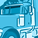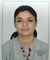# New to Qlik Sense

If you’re new to Qlik Sense, start with this Discussion Board and get up-to-speed quickly.

Announcements
WE ARE LISTENING! New Navigation for Qlik Community, Sept. 26: TELL ME MORE
cancel
Showing results for
Did you mean:Contributor II

## Compare 2 tables and show how similar they are

Hi,

I have a bill of materials tables that will contain the following key pieces of information.

1. BOM Code

2. Stock Code

Each BOM will have one or more stock codes. Example data as below

 BOM Code Stock Code A1 WH1 A1 BH4 A1 OP3 A2 OP3 A2 BH4 A2 ZA6

I am trying to create a way to select a single BOM Code and have Qlik compare all of the other BOM codes to show how similar they are.  The first way of doing this is to show how many common ingredients there are.

So far I have filed in trying to use alternate states with the P function and I have also failed in trying to use two tables in the load joined by the common stock codes.

I am trying to get to a simple formula like the pseudocode below.

Count([Compared Common BOM Stock Code]) / Count([Selected BOM Stock Code])  = Similarity Measure

So for the table above I'd have A1 = 3 and A2 = 2 i.e. 2/3 = 0.66

It seems like it should be fairly straightforward but so far a viable solution has alluded me.

Any help appreciated.

Labels (5)

• ### values

3 RepliesCreator II

U need to do the Intersection between Compared and Selected BOM Code:

May be Something Like This

count({<[Stock Code]=p({<[BOM Code]={'\$(=only([BOM Code]))'}>}[Stock Code])>*<[Stock Code]=p({<[BOM Code]={'\$(=only({G1}[BOM Code]))'}>}[Stock Code])>}Distinct [Stock Code])/
count({<[BOM Code]={'\$(=only([BOM Code]))'}>}Distinct [Stock Code])

where G1 is the Alternate State.

let me know if this help

Thanks,

PriyankaContributor II
Author

Hi Priyanka,

Thanks for this.

Can you explain how this would be setup on screen so that the alternate state is used?

I'm trying to visualise it so that I can configure the objects properly and I'm not sure if I have followed your line of thinking.

ThanksCreator II

Basically its a intersection of selected and compared set of records

If u have selected A1 -BOM code : default state

And u have selected A2 - BOM code : Alternate state(G1)

Then above mentioned formula would work...

<Stock code of selected BOM code(default state) >*<Stock code of compared BOM code(alternate state)>/Stock code of selected BOM code(default state

Hope this helps.

Thanks

Priyanka